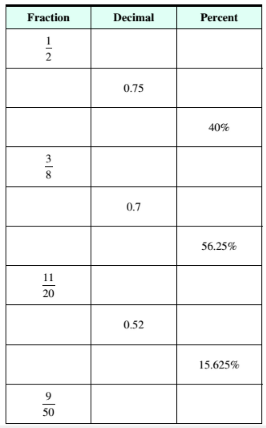Chapter 9.3, Problem 4ESMathematical Excursions (MindTap C...

4th Edition
Richard N. Aufmann + 3 others
ISBN: 9781305965584

Solutions

Chapter
SectionMathematical Excursions (MindTap C...

4th Edition
Richard N. Aufmann + 3 others
ISBN: 9781305965584
Textbook Problem

Complete the table of equivalent fractions, decimals, and percent’s.To determine

To calculate: The fraction and percent for the decimal 0.75 .

Explanation

Given Information:

Decimal, 0.75

Formula used:

Steps to write decimal as fraction,

Step I

: Calculate the percent value of the decimal.

Step II

: Remove the percent sign of the percentage value and multiply the number by 1100 .

Step III: Simplify the fraction.

Calculation:

Consider the decimal,

0.75

Move the decimal point two places to the right and place a percent sign, to write decimal as percent,

0.75=75%

Thus, the percent value of decimal 0.75 is 75% .

Now, remove the percent sign from the percentage value and multiply it by 1100

Still sussing out bartleby?

Check out a sample textbook solution.

See a sample solution

The Solution to Your Study Problems

Bartleby provides explanations to thousands of textbook problems written by our experts, many with advanced degrees!

Get Started

In problems 31-36, simplify each expression. 33.

Mathematical Applications for the Management, Life, and Social Sciences

Find y" if x6+y6=1.

Calculus (MindTap Course List)

16x4y84

Applied Calculus for the Managerial, Life, and Social Sciences: A Brief Approach

Convert the expressions in Exercises 6584 to power form. 2x3x0.12+43x1.1

Finite Mathematics and Applied Calculus (MindTap Course List)

Evaluate the integral, if it exists. 0T(x48x+7)dx

Single Variable Calculus: Early Transcendentals